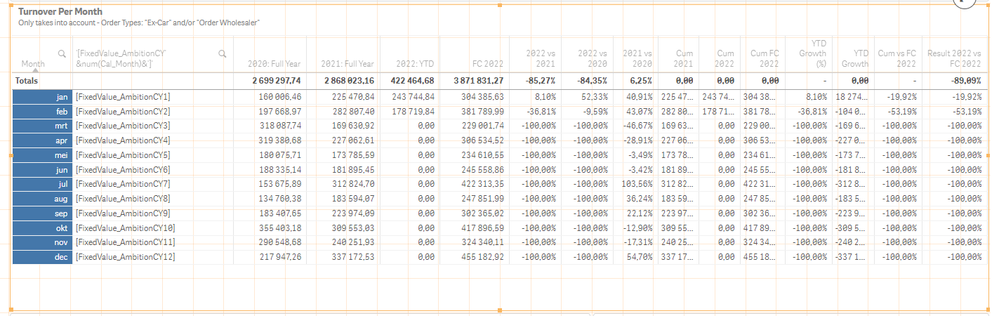# New to Qlik Sense

If you’re new to Qlik Sense, start with this Discussion Board and get up-to-speed quickly.

Announcements
QlikWorld 2023, a live, in-person thrill ride. Save \$300 before February 6: REGISTER NOW!
cancel
Showing results for
Did you mean:Contributor III

## Concatenate to Reference an Existing Field Name - Turn String into Field Name

Good morning

I have a pivot table that takes month as a dimension (Cal_Month), the rest are measures to calculate turnover per month, vs, %, ... .

However, I need to add an additional column, to show the fields: FixedValue_AmbitionCY1, FixedValue_AmbitionCY2, FixedValue_AmbitionCY3, ...

The last number of these fields corresponds with the num(Cal_Month). Jan is num(Cal_Month) = 1 so FixedValue_AmbitionCY1 should be shown. For Feb that should be FixedValue_AmbitionCY2 and zo on.

I tried with this simple formula but it just shows it as a string, not as a field. How can I change this so that Qlik sees it as a field name and not as a string?

='[FixedValue_AmbitionCY' &num(Cal_Month)&']'Labels (4)

• ### Variables

1 Solution

Accepted SolutionsContributor III
Author

It's not the cleanest solution, and does not take into account if there would be more Cal_Month added (thankfully it's safe to say we'll never have more than 12 months in a year), but if it works it works!

If used for fields where there will be more added values, this solution requires maintenance:

IF ( num(Cal_Month) = '1', FixedValue_AmbitionCY1,

IF ( num(Cal_Month) ='2', FixedValue_AmbitionCY2,

IF ( num(Cal_Month) ='3', FixedValue_AmbitionCY3,

IF ( num(Cal_Month) ='4', FixedValue_AmbitionCY4,

IF ( num(Cal_Month) ='5', FixedValue_AmbitionCY5,

IF ( num(Cal_Month) ='6', FixedValue_AmbitionCY6,

IF ( num(Cal_Month) ='7', FixedValue_AmbitionCY7,

IF ( num(Cal_Month) ='8', FixedValue_AmbitionCY8,

IF ( num(Cal_Month) ='9', FixedValue_AmbitionCY9,

IF ( num(Cal_Month) ='10', FixedValue_AmbitionCY10,

IF ( num(Cal_Month) ='11', FixedValue_AmbitionCY11,

IF ( num(Cal_Month) ='12', FixedValue_AmbitionCY12

) //end if num(Cal_Month) = '12'

) //end if num(Cal_Month) = '11'

) //end if num(Cal_Month) = '10'

) //end if num(Cal_Month) = '9'

) //end if num(Cal_Month) = '8'

) //end if num(Cal_Month) = '7'

) //end if num(Cal_Month) = '6'

) //end if num(Cal_Month) = '5'

) //end if num(Cal_Month) = '4'

) //end if num(Cal_Month) = '3'

) //end if num(Cal_Month) = '2'

) //end if num(Cal_Month) = '1'Contributor III
Author

It's not the cleanest solution, and does not take into account if there would be more Cal_Month added (thankfully it's safe to say we'll never have more than 12 months in a year), but if it works it works!

If used for fields where there will be more added values, this solution requires maintenance:

IF ( num(Cal_Month) = '1', FixedValue_AmbitionCY1,

IF ( num(Cal_Month) ='2', FixedValue_AmbitionCY2,

IF ( num(Cal_Month) ='3', FixedValue_AmbitionCY3,

IF ( num(Cal_Month) ='4', FixedValue_AmbitionCY4,

IF ( num(Cal_Month) ='5', FixedValue_AmbitionCY5,

IF ( num(Cal_Month) ='6', FixedValue_AmbitionCY6,

IF ( num(Cal_Month) ='7', FixedValue_AmbitionCY7,

IF ( num(Cal_Month) ='8', FixedValue_AmbitionCY8,

IF ( num(Cal_Month) ='9', FixedValue_AmbitionCY9,

IF ( num(Cal_Month) ='10', FixedValue_AmbitionCY10,

IF ( num(Cal_Month) ='11', FixedValue_AmbitionCY11,

IF ( num(Cal_Month) ='12', FixedValue_AmbitionCY12

) //end if num(Cal_Month) = '12'

) //end if num(Cal_Month) = '11'

) //end if num(Cal_Month) = '10'

) //end if num(Cal_Month) = '9'

) //end if num(Cal_Month) = '8'

) //end if num(Cal_Month) = '7'

) //end if num(Cal_Month) = '6'

) //end if num(Cal_Month) = '5'

) //end if num(Cal_Month) = '4'

) //end if num(Cal_Month) = '3'

) //end if num(Cal_Month) = '2'

) //end if num(Cal_Month) = '1'Tags
Community Browser